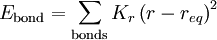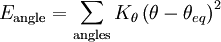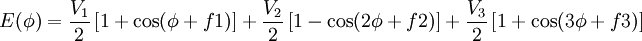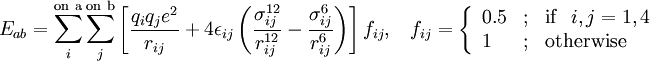# OPLS force field

(diff) ← Older revision | Latest revision (diff) | Newer revision → (diff)This article is a 'stub' page, it has no, or next to no, content. It is here at the moment to help form part of the structure of SklogWiki. If you add sufficient material to this article then please remove the {{Stub-general}} template from this page.

The optimized potentials for liquid simulations (OPLS) force-field   was developed for the simulation of proteins and other organic liquids.

## OPLS-all atom

(OPLS-AA)  

#### Form of the force field

Bond stretching$E_{\rm {bond}} = \sum_{\rm {bonds}} K_r \left(r-r_{eq}\right)^2$

Angle bending$E_{\rm {angle}} = \sum_{\rm {angles}} K_\theta \left(\theta-\theta_{eq}\right)^2$

Torsion$E(\phi) = \frac{V_1}{2} \left[ 1 + \cos (\phi +f1)\right] + \frac{V_2}{2} \left[ 1 - \cos (2\phi +f2)\right] + \frac{V_3}{2} \left[ 1 + \cos (3\phi +f3)\right]$

Non-bonded$E_{ab} = \sum_i^{\rm {on~a}}\sum_j^{\rm {on~b}} \left[ \frac{q_i q_j e^2}{r_{ij}} + 4\epsilon_{ij} \left( \frac{\sigma_{ij}^{12}}{r_{ij}^{12}}-\frac{\sigma_{ij}^{6}}{r_{ij}^{6}}\right)\right]f_{ij}, ~~~~f_{ij} = \left\{ \begin{array}{lll} 0.5 & ; & {\rm {if}}~~ i,j = 1,4 \\ 1 & ; & {\rm {otherwise}} \end{array} \right.$